## Solution to 1986 Problem 21

 The invariant interval is defined by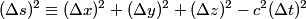\begin{align*}(\Delta s)^2 \equiv (\Delta x)^2 + (\Delta y)^2 + (\Delta z)^2 - c^2(\Delta t)^2\end{align*}Plug in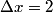$\Delta x = 2$,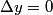$\Delta y = 0$,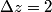$\Delta z = 2$,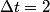$\Delta t = 2$, and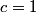$c = 1$ to find that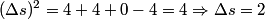\begin{align*}(\Delta s)^2 = 4 + 4 + 0 - 4 = 4 \Rightarrow \Delta s = 2\end{align*}Hence answer (C) is correct.